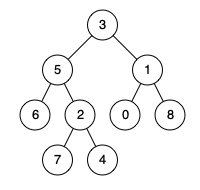| English | 简体中文 |

# 236. Lowest Common Ancestor of a Binary Tree

## Description

Given a binary tree, find the lowest common ancestor (LCA) of two given nodes in the tree.

According to the definition of LCA on Wikipedia: “The lowest common ancestor is defined between two nodes p and q as the lowest node in T that has both p and q as descendants (where we allow a node to be a descendant of itself).”

Example 1:Input: root = [3,5,1,6,2,0,8,null,null,7,4], p = 5, q = 1
Output: 3
Explanation: The LCA of nodes 5 and 1 is 3.


Example 2:Input: root = [3,5,1,6,2,0,8,null,null,7,4], p = 5, q = 4
Output: 5
Explanation: The LCA of nodes 5 and 4 is 5, since a node can be a descendant of itself according to the LCA definition.


Example 3:

Input: root = [1,2], p = 1, q = 2
Output: 1


Constraints:

• The number of nodes in the tree is in the range [2, 105].
• -109 <= Node.val <= 109
• All Node.val are unique.
• p != q
• p and q will exist in the tree.